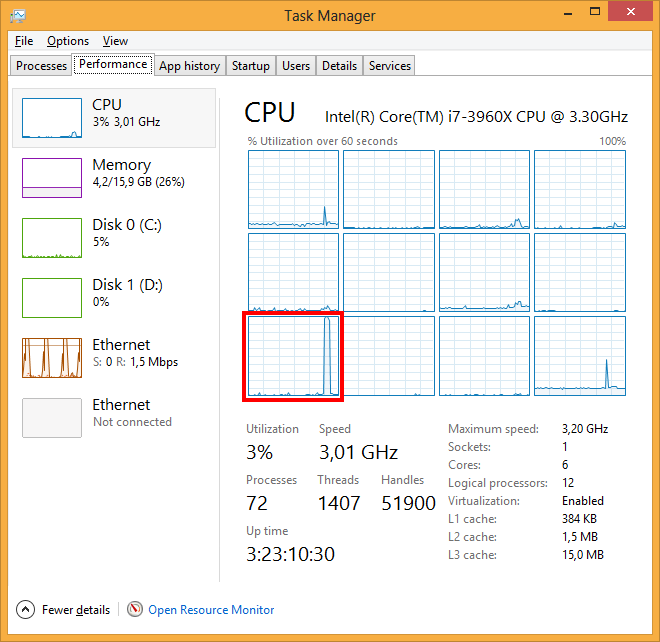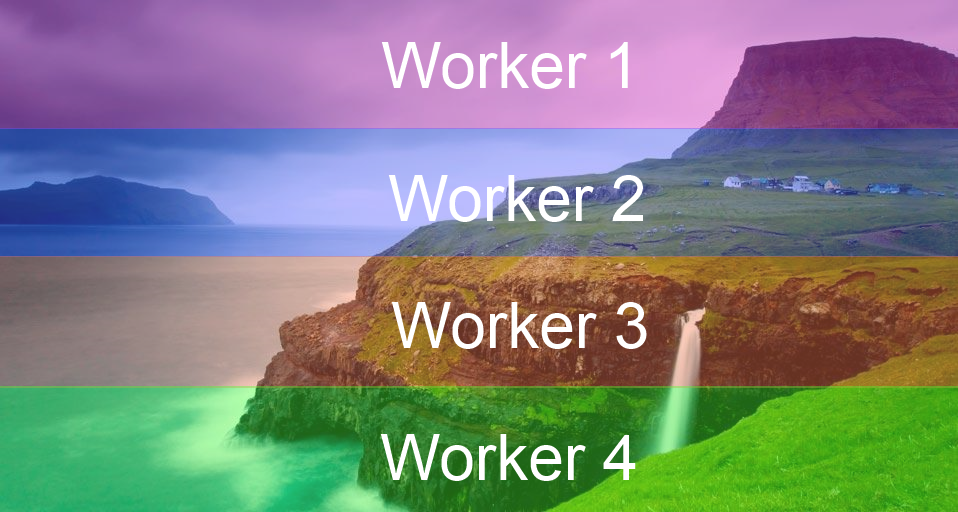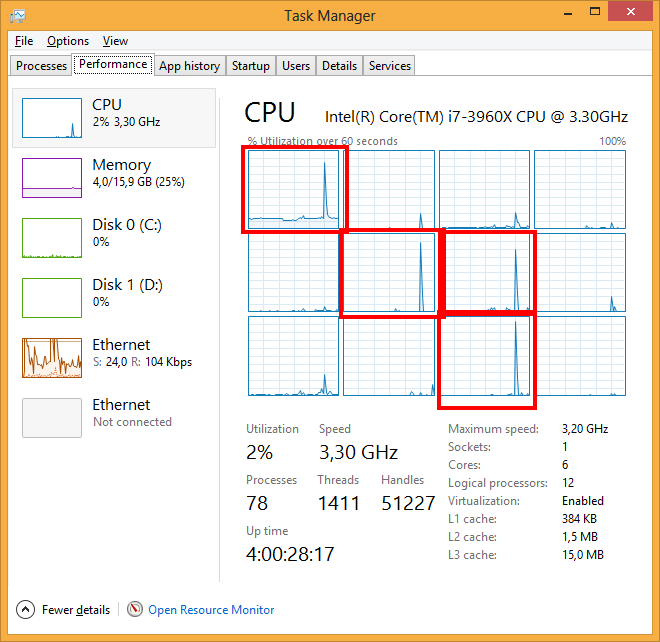# How to Use Web Workers for Image Manipulation

Posted · Category: Information

Today I’d like to talk about picture manipulation in HTML5, using pure Javascript.

### The Test Case

The test application is simple. On the left a picture to manipulate and on the right the updated result (a sepia tone effect is applied):The page itself is simple and is described as follow:

```<!DOCTYPE html>
<html>
<meta charset="utf-8" />
<title>PictureWorker</title>

<body id="root">
<div id="sourceDiv">
<img id="source" src="mop.jpg" />
</div>
<div id="targetDiv">
<canvas id="target"></canvas>
</div>
<div id="log"></div>
</body>
</html>
```

The overall process to apply a sepia tone effect requires you to compute a new RGB value for every pixel of the existing source picture, and then render it on the <canvas> tag with id=”target”. Below are the formulae we use to create the new RGB values from the existing RGB values of a pixel:

finalRed= (red * 0.393) + (green * 0.769) + (blue * 0.189);
finalGreen = (red * 0.349) + (green * 0.686) + (blue * 0.168);
finalBlue= (red * 0.272) + (green * 0.534) + (blue * 0.131);

To make it a bit more realistic, I’ve added a bit of randomness to my sepia formulae. I create a noise value which ranges from 0.5 to 1, which determines the degree to which my final pixel output matches the RGB value calculated via the formulae above, versus the degree to which it retains it’s original RGB values.

```function noise() {
//Returns a value between 0.5 and 1
return Math.random() * 0.5 + 0.5;
};

function colorDistance(scale, dest, src) {
// returns a red, blue or green value for the 'sepia' pixel
// which is a weighted average of the original value and the calculated value
return (scale * dest + (1 - scale) * src);
};

var processSepia = function (pixel) {
// takes a given pixel and updates its red, blue and green values
// using a randomly weighted average of the initial and calculated red/blue/green values
pixel.r = colorDistance(noise(), (pixel.r * 0.393) + (pixel.g * 0.769) + (pixel.b * 0.189), pixel.r);
pixel.g = colorDistance(noise(), (pixel.r * 0.349) + (pixel.g * 0.686) + (pixel.b * 0.168), pixel.g);
pixel.b = colorDistance(noise(), (pixel.r * 0.272) + (pixel.g * 0.534) + (pixel.b * 0.131), pixel.b);
};```

### Brute force

Obviously the very first solution consists of using brute force, with a function that apply the previous code on every pixel. To get access to the pixels, you can use the canvas context with the following code, which creates a pointer to the source img and the target canvas, and :

```var source = document.getElementById("source");

var canvas = document.getElementById("target");
canvas.width = source.clientWidth;
canvas.height = source.clientHeight;

// ... tempContext is the 2D context of canvas
tempContext.drawImage(source, 0, 0, canvas.width, canvas.height);

var canvasData = tempContext.getImageData(0, 0, canvas.width, canvas.height);
var binaryData = canvasData.data;
}```

At this point, the binaryData object contains an array of every pixel and can be used to quickly read or write data directly to the canvas. With this in mind, we can apply the whole effect with the following code:

```    var source = document.getElementById("source");

var start = new Date();

var canvas = document.getElementById("target");
canvas.width = source.clientWidth;
canvas.height = source.clientHeight;

if (!canvas.getContext) {
log.innerText = "Canvas not supported. Please install a HTML5 compatible browser.";
return;
}

var tempContext = canvas.getContext("2d");
// len is the number of items in the binaryData array
// it is 4 times the number of pixels in the canvas object
var len = canvas.width * canvas.height * 4;

tempContext.drawImage(source, 0, 0, canvas.width, canvas.height);

var canvasData = tempContext.getImageData(0, 0, canvas.width, canvas.height);
var binaryData = canvasData.data;

// processSepia is a variation of the previous version. See below
processSepia(binaryData, len);

tempContext.putImageData(canvasData, 0, 0);
var diff = new Date() - start;
log.innerText = "Process done in " + diff + " ms (no web workers)";

}```

The processSepia function is just an variation of the previous one:

```var processSepia = function (binaryData, l) {
for (var i = 0; i < l; i += 4) {
var r = binaryData[i];
var g = binaryData[i + 1];
var b = binaryData[i + 2];

binaryData[i] = colorDistance(noise(), (r * 0.393) + (g * 0.769) + (b * 0.189), r);
binaryData[i + 1] = colorDistance(noise(), (r * 0.349) + (g * 0.686) + (b * 0.168), g);
binaryData[i + 2] = colorDistance(noise(), (r * 0.272) + (g * 0.534) + (b * 0.131), b);
}
};```

With this solution, on my Intel Extreme processor (12 cores), the main process takes 150ms and obviously only use one processor:### Enter web workers

The best thing you can do when dealing with SIMD (single instruction multiple data) is to use a parallelization approach. Especially when you want to work with low-end hardware (such as phone devices) with limited resources.

In JavaScript, to enjoy the power of parallelization, you have to use the Web Workers. My friend David Rousset wrote an excellent paper on this subject.

Picture processing is a really good candidate for parallelization because (in the case of our sepia tone function)every processing is independent. Therefore, the following approach is possible:To do so, first of all you have to create a tools.js file to be used as a reference by other scripts.

```// add the below functions to tools.js
function noise() {
return Math.random() * 0.5 + 0.5;
};

function colorDistance(scale, dest, src) {
return (scale * dest + (1 - scale) * src);
};

var processSepia = function (binaryData, l) {
for (var i = 0; i < l; i += 4) {
var r = binaryData[i];
var g = binaryData[i + 1];
var b = binaryData[i + 2];

binaryData[i] = colorDistance(noise(), (r * 0.393) + (g * 0.769) + (b * 0.189), r);
binaryData[i + 1] = colorDistance(noise(), (r * 0.349) + (g * 0.686) + (b * 0.168), g);
binaryData[i + 2] = colorDistance(noise(), (r * 0.272) + (g * 0.534) + (b * 0.131), b);
}
};
```

The main point of this script is that a portion of the canvas data, namely the part required for the current block to process, is cloned by JavaScript and passed to the worker. The worker is not working on the initial source but on a copy of it (using the structured clone algorithm). The copy itself is really quick and limited to a specific part of the picture.

The main client page (default.js) has to create 4 workers and give them the right part of the picture. Then every worker will callback a function in the main thread using the messaging API (postMessage / onmessage) to give back the result:

```var source = document.getElementById("source");

// We use var start at the beginning of the code and stop at the end to measure turnaround time

var start = new Date();

var canvas = document.getElementById("target");
canvas.width = source.clientWidth;
canvas.height = source.clientHeight;

// Testing canvas support
if (!canvas.getContext) {
log.innerText = "Canvas not supported. Please install a HTML5 compatible browser.";
return;
}

var tempContext = canvas.getContext("2d");
var len = canvas.width * canvas.height * 4;

// Drawing the source image into the target canvas
tempContext.drawImage(source, 0, 0, canvas.width, canvas.height);

// If workers are not supported
// Perform all calculations in current thread as usual
if (!window.Worker) {
// Getting all the canvas data
var canvasData = tempContext.getImageData(0, 0, canvas.width, canvas.height);
var binaryData = canvasData.data;

// Processing all the pixel with the main thread
processSepia(binaryData, len);

// Copying back canvas data to canvas
tempContext.putImageData(canvasData, 0, 0);

var diff = new Date() - start;
log.innerText = "Process done in " + diff + " ms (no web workers)";

return;
}

// Let say we want to use 4 workers
// We will break up the image into 4 pieces as shown above, one for each web-worker
var workersCount = 4;
var finished = 0;
var segmentLength = len / workersCount; // This is the length of array sent to the worker
var blockSize = canvas.height / workersCount; // Height of the picture chunck for every worker

// Function called when a job is finished
var onWorkEnded = function (e) {
// Data is retrieved using a memory clone operation
var canvasData = e.data.result;
var index = e.data.index;

// Copying back canvas data to canvas
// If the first webworker  (index 0) returns data, apply it at pixel (0, 0) onwards
// If the second webworker  (index 1) returns data, apply it at pixel (0, canvas.height/4) onwards, and so on
tempContext.putImageData(canvasData, 0, blockSize * index);

finished++;

if (finished == workersCount) {
var diff = new Date() - start;
log.innerText = "Process done in " + diff + " ms";
}
};

// Launching every worker
for (var index = 0; index < workersCount; index++) {
var worker = new Worker("pictureProcessor.js");
worker.onmessage = onWorkEnded;

// Getting the picture
var canvasData = tempContext.getImageData(0, blockSize * index, canvas.width, blockSize);

// Sending canvas data to the worker using a copy memory operation
worker.postMessage({ data: canvasData, index: index, length: segmentLength });
}
};

source.src = "mop.jpg";```

Using this technique, the complete process lasts only 80ms (from 150ms) on my computer and obviously uses 4 processors:On my low-end hardware (based on dual core system), the process time goes down to 500ms (from 900ms).

The final code can be downloaded here, and a working example is posted here. For comparison, here is the same code without web workers.

A important point to note is that on recent computers the difference can be small, or even in favor of the code without workers. The overhead of the memory copy must be balanced by a complex code used by the workers. The above example of a sepia tone conversion might not be enough in some cases to warrant switching to web workers.
However, the web workers will really be useful on low-end hardware with multiple cores.

### Porting to Windows 8

Finally I was not able to resist to the pleasure of porting my JavaScript code to create a Windows 8 application. It took me about 10 minutes to create a blank JavaScript project and copy/paste the JavaScript code inside. You can grab the Windows app code here, and feel the power of native JavaScript code for Windows 8!

David Catuhe is a Technical Evangelist Lead at Microsoft France, specializing in HTML5 and web development. This article originally appeared on his MSDN blog, EternalCoding on 20th September, 2012.You can follow him @deltakosh on Twitter.

Search